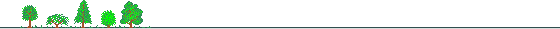Appendix: Methodology for Compilation of Multifactor Productivity Statistics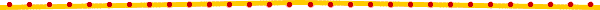Compilation of Capital Productivity Statistics

1. Estimation of Capital Stock:

The approaches used to estimate capital stock are listed as follows:

 Direct Estimation Method - Perpetual Inventory Method - Benchmark-year Method - Benchmark Extrapolation Method - Average Depreciation Method - Polynominal-benchmark Method Indirect Estimation Method - Estimation by Production Function and Capital Stock at the Same Time - Estimation by Investment Function and Depreciation Patterm at the Same Time

In this report, the benchmark extrapolation method is adopted to estimate the capital stock, with the benchmark-year being designated as those when each industrial and commercial census took place and 1988 when the national wealth census was conducted. Please see the "Study on Statistical Measurement of Capital Productivity in Taiwan Area", published by the DGBAS, for details of the estimation method. Key points of the method are briefly introduced as follows:

 (1) Estimation of Gross Fixed Capital Stock: GK(t) = GK(t-1) + I(t) where GK(t) refers to the amount of fixed capital stock in t period. (2) Estimation of Net Fixed Capital Stock: NK(t) = NK(t-1) + I(t) - D(t) where GK(t) refers to the amount of fixed capital stock in t period, I(t) refers to the amount of real gross fixed capital formation in t period, D(t) refers to real fixed capital depreciation in t period.

2. Amount of Fixed Capital in Actual Utilization:

 FGK(t) = f * GK(t) FNK(t) = f * NK(t) where FGK(t) refers to the amount of gross fixed capital actually utilized in t period, FNK(t) refers to the amount of net fixed capital actually utilized in t period, and f refers to the utilization rate of equpiment.

3. Mid-Year Real Fixed Capital Stock:

gK(t) = [GK(t) + GK(t-1)]/2

nK(t) = [NK(t) + NK(t-1)]/2

 where gK(t) refers to mid-year real gross fixec capital stock in t period, and nK(t) refers to mid-year real net fixed capital stock in t period.

4. Fixed Capital Productivity:

 (1) Gross Fixed Capital Productivity: GKP = GDPR/gK(t) GKPI = GDPIR/gK(t)I
 where GKP refers to gross fixed capital productivity, GKPI refers to index for gross fixed capital productivity, GDPR refers to real gross domestic product, GDPIR refers to index for gross domestic product, and gK(t)I refers to index for mid-year real gross fixed capital stock in t period.
 (2) Net Fixed Capital Productivity: NKP = NDPR/nK(t) NKPI = NDPIR/nK(t)I
 where NKP refers to net fixed capital productivity, NKPI refers to index for net fixed capital productivity, NDPR refers to real net domestic product, NDPIR refers to index for net domestic product, and NK(t)I refers to index for mid-year real net fixed capital stock in t period.

Compilation of Multifactor Productivity

1. Estimation of Production Function Model:

In this report, the production function model is built on the base of a general, widely used translog production function which is characterized by non-fixed substitute elasticity and which is, therefore, subject to less constraints than a general logarithm liner model. For the reason, this production function model could be used in a wide range of applications.

2. Estimation for Input Amount of Each Production Factor:

In this report, the production factors are mainly capital stock and total work hours of the employment for which data are obtained from the "Study on Statistical Measurement of Capital Productivity in Taiwan Area" and "The Trend in Labor Productivity in Taiwan Area" both publications are published by the DGBAS.

3. Computation of Percentage Share for Each Input Factor:

This involves calculation of the labor compensation and the capital compute cost. As the total of the capital's percentage share and the labor's percentage share equals to one, the only job is to calculate the percentage share for labor. The percentage share for capital can be derived by deducting one by the percentage share for labor.

 Based on the above-mentioned theoretical framework, the total cost can be defined as follows: Total factor Cost = Nominal Output (PQ) = Gross Domestic Product (GDP) - Indirect Tax + Subsidy

In principle, the labor compensation is defined as the adjusted compensation for empolyment population, i.e., compensation for employees plus the average compensation per person multiplied by the difference between the number of employment population and the number of employees.

The equations are listed below: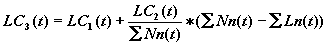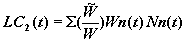where LC1(t) refers to compensation for employment as shown in national income accounts, LC2(t) refers to compensation for employment population, Ln(t) refers to the number of employees each month,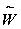(t) refers to the average compensation per person in a certain month, W(t) refers to the average wage per person in a certain month, Wn(t) refers to the wage per person in each month, and Nn(t) refers to the employment population in each month.

The percentage share for labor is derived by dividing the labor compensation into the total cost (nominal output). As some data vary in coverage for different industries, appropriate adjustment is needed. For instance, for the manufacturing, construction industries and service sector, the labor compensation is obtained from the compensation for employees as shown in the national income statistics.

4. Measurement of Real Output:

The real output here refers to the ral gross domestic product which is used in computation of the multifactor productivity index. In this report, data for real output index are obtained from the "The Trend in Labor Productivity in Taiwan Area," published by the DGBAS.

5. Computation of Total Factor Input Index:

The Tornqvist input index is

I(t): Tornqvist total input index.

 ln(I(t)/I(t-1)) =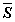Kt * ln(K(t)/K(t-1)) + =Lt * ln(L(t)/L(t-1))

where L and K denotes labor and capital inputs, respectively; SL and SK denotes the percentage shares for labor and capital, respectively; t refers to time;Kt = (Kt +Kt-1)/2Lt = (Lt +Lt-1)/2

The base year is first given a value of 100. Then the data derived from items 2 and 3 are fed into the Tornqvist input index formula.

6. Computation of Multifactor Productivity Index:

First, the real gross domestic product Index is divided by the total factor input index. Second, the results are adjusted to become the desired by giving 100 as the value of the base year.